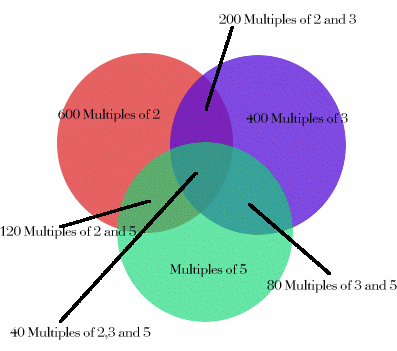#### You may also likeMake a set of numbers that use all the digits from 1 to 9, once and once only. Add them up. The result is divisible by 9. Add each of the digits in the new number. What is their sum? Now try some other possibilities for yourself!### Have You Got It?

Can you explain the strategy for winning this game with any target?### Counting Factors

Is there an efficient way to work out how many factors a large number has?

# Inclusion Exclusion

##### Age 11 to 14Challenge Level

How many integers between 1 and 1200 are NOT multiples of any of the numbers 2, 3 or 5?

Venn diagram showing whole numbers from 1 to 1200Working along the same lines, Emma and Laura from The Mount School in York sent the diagram above, and Lorn from Stamford School sent the solution below:

Simplify the problem by trying 1-30 (because 2 x 3 x 5 = 30 and 30 is a factor of 1200).

First I crossed off all the multiples of 2 (even numbers). Then I crossed off all multiples of 3, then all multiples of 5. I then counted 8 numbers which were not crossed off.

I tried 31-60 and found a pattern.
Comparing 31-60 and 1-30, the crossed off numbers corresponded.
This is not surprising since you get the numbers in the second set just by adding 30 to the numbers in the first set.
I also noticed that the numbers I did not cross off were prime numbers and 2, 3 and 5 are prime.

There are 40 sets of 30 numbers from 1 to 1200 (1-30, 31-60, 61-90 ... ).
From this I conclude that in each set there are 8 numbers that are not multiples of 2, 3 or 5, so there are 8 x 40 = 320 numbers altogether that are not multiples of 2, 3 or 5.

Lorn found another method, using similar ideas, sparked from the Venn diagram shown in the original question:

The diagram shows three sets of numbers: multiples of 2, multiples of 3 and multiples of 5.
When counting all the numbers in the three sets (600 + 400 + 240 = 1240) some numbers are counted twice and some numbers are counted 3 times.
Deducting the number of 'double counted' numbers we get 1240 - 200 - 80 - 120 = 840. Now the 'triple counted' numbers have been removed 3 times so we need to add on 40 to include them, giving 880 numbers which are multiples of one or more of the given numbers.
It follows that 1200 - 880 = 320 numbers are not multiples of any of these numbers.

Congratulations for your work on this problem to Samantha of Hethersett High School, Norfolk, Jenny, Caroline, Emma, Rachel and Beth from the Mount School, York and to Ben.